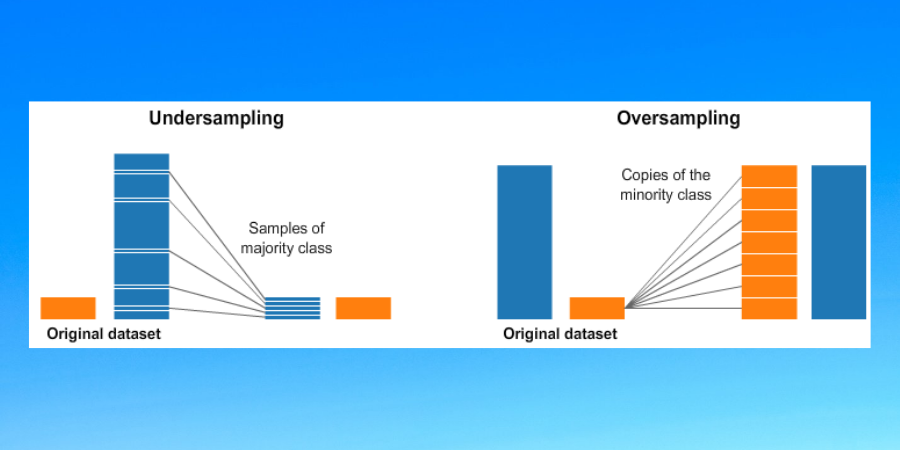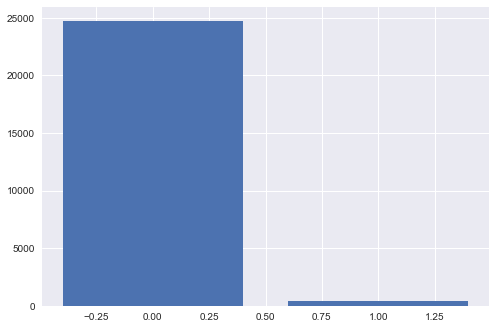# Handling Imbalanced DatasetsS.No Lesson Title
1
2
3
##### Methods to Tackle Imbalanced Class Problem
3.1 Performance Metric
3.2 Random Under Sampling
3.3 Random Oversampling
3.4 Hyperparameters
3.5 Using Tree-Based Models
3.6 Synthetic Minority Oversampling Technique(SMOTE)
4

### Introduction

Accuracy is a common metric that comes to everyone's mind when we talk about the performance of a model or algorithm on a classification task. But accuracy is not a good metric when we deal with imbalanced data where the distribution of the data points belonging to the classes are not equal sampled or distributed. Imbalanced data problems are commonly seen in the case of classification tasks, image segmentation and need to be addressed at the start of the model-building stage itself. If not taken care of it can lead to models with a large bias towards a particular class. We'll talk more about this in the upcoming sections along with methods to solve imbalance class classification tasks. Let's get started.

### Overview of Imbalanced Class Problem

We all have seen problems where one class has more observations than other classes. This can happen due to many reasons including the rarity of observations of one class, ease of capturing data for a class, etc. Some common examples include fraud detection, cancer detection, spam filtering, advertising clicks, etc. Have a look at the following bar graph drawn using a fraud detection dataset:You can clearly see that the number of fraud classes is very less and such exploratory analysis is very crucial for every classification task. Imbalanced data can hamper the prediction ability of our model resulting in accuracy values that are not credible. This happens because our model can simply predict the majority class every time and get higher accuracy but on most occasions, our purpose is to capture observations from minority class which is not fulfilled here. This happens with imbalanced datasets as most of the algorithms are developed to maximize accuracy and reduce errors. In the upcoming section, we'll have a look at different methods to tackle imbalanced class datasets.

### Methods to tackle Imbalanced Class Problem

There are many ways to handle the imbalanced class problem and we'll start with some common mistakes done while facing imbalanced class problems:

#### 1. Performance Metric

This arguably the most common error done by beginners as they tend to ignore the fact that accuracy is not the suitable metric while dealing with imbalanced class problems. What can go wrong is that the classifier can always predict the frequently appearing class leading to an accuracy score that's high. This issue can be easily resolved by using other metrics such as precision, recall, f1 score, Area Under ROC curve, confusion matrix, etc. We won't go into the details of these metrics in this section but the following is a code snippet to implement roc_auc metric (assume clf is our classification model, X is input feature vector, y is class probabilities):

``````from sklearn.metrics import roc_auc_score

pred_y = clf.predict_proba(X)
roc_auc_score(y, pred_y)
``````

#### 2. Random Under Sampling

This method can be used when we have large amounts of data and removing some rows might not lead to loss of information. In this method, some observations from the majority class are removed to reduce the imbalance in the dataset to some extent. Have a look at the following code snippet for the same (assume 'data' is a pandas data frame having class label 0 and 1 in column 'class', also assume 0 label corresponds to minority class):

```from sklearn.utils import resample

#separate class
class_0 = data[data['Class']]== 0]
class_1 = data[data['Class']]== 1]
df_under_1 = resample(class_1,
replace=True,
n_samples=n_samples_after_resampling
)
df_undersampled = pd.concat(
[df_under_1,class_0]
)
```

#### 3. Random Over Sampling

This method is used to increase the observations of the minority classes. There are many ways of doing this but the simplest is by randomly duplicating observations with replacement. Following is the code snippet to do so:

```from sklearn.utils import resample

#separate class
class_0 = data[data['Class']]== 0]
class_1 = data[data['Class']]== 1]
df_over_0 = resample(
class_0,
replace=True,
n_samples=n_samples_after_resampling
)
df_oversampled = pd.concat(
[df_over_0, class_1]
)
```

#### 4. Hyperparameters

Many classification algorithms come with hyperparameters that can be set equal to the ratio classes (only for binary classification problems) or to some other value which tells the model that the dataset is imbalanced. For example, in tree-based models, the hyperparameter scal_pos_weight can be set to a ratio of minority to majority classes for imbalanced data. These hyperparameters then add some weight to observations of undersampled class so the model doesn't overlook them while training. Following is an example for the same where we set class_weight of SVM to 'balanced' to tell out model that our dataset is not imbalanced:

``````from sklearn.svm import SVC

clf = SVC(
kernel='rbf',
class_weight = 'balanced',
probability = True
)
``````

#### 5. Using Tree-Based Models

It has been shown that tree-based models have a really good performance while handling imbalanced datasets. This happens because they have a hierarchical structure where each split is done based on some feature enabling the trees to learn more about each feature and class even in the case of the imbalanced dataset. When we talk about tree-based models we often talk about ensembles as ensembles outperform a single tree in every use case. Ensemble models like the random forest, gradient boosting trees are very popular tree-based models. Let's have a look at an ensemble model:

``````from xgboost import XGBClassifier

clf = XGBClassifier(
n_iterators=200,
learning_rate = 0.05,
scal_pos_weight = rate_of_minortiy_to_majority_class,
probability = True
)
``````

#### 6. Synthetic Minority Oversampling Technique (SMOTE)

This technique uses a KNN based algorithm to select some neighboring points and then sets up some synthetic data points between these neighbors. Following is a step by step explanation of how it works:

1. Take the input and identify the minority class.
2. Find K nearest neighbors of a point in the minority class. (number of neighbors to be taken can be specified using k_neighbors hyperparameter)
3. Now take this point and the neighbors and start placing synthetic points on a line between the point under consideration and its neighbor.
4. Repeat the above steps by choosing a new point from the minority space each time.

Following is a code snippet for the same:

``````from imblearn.over_sampling import SMOTE
sm = SMOTE()

#getting oversampled datasets from original datasets
x_smote, y_smote = sm.fit_resample(x,y)
``````

### Conclusion

With this, we come to the end of this article. We have tried to explain different techniques to handle imbalanced datasets. There are many more techniques but the ones mentioned in this article are the most effective and should be tried before other techniques out there. Happy Learning!

Send Message
Top